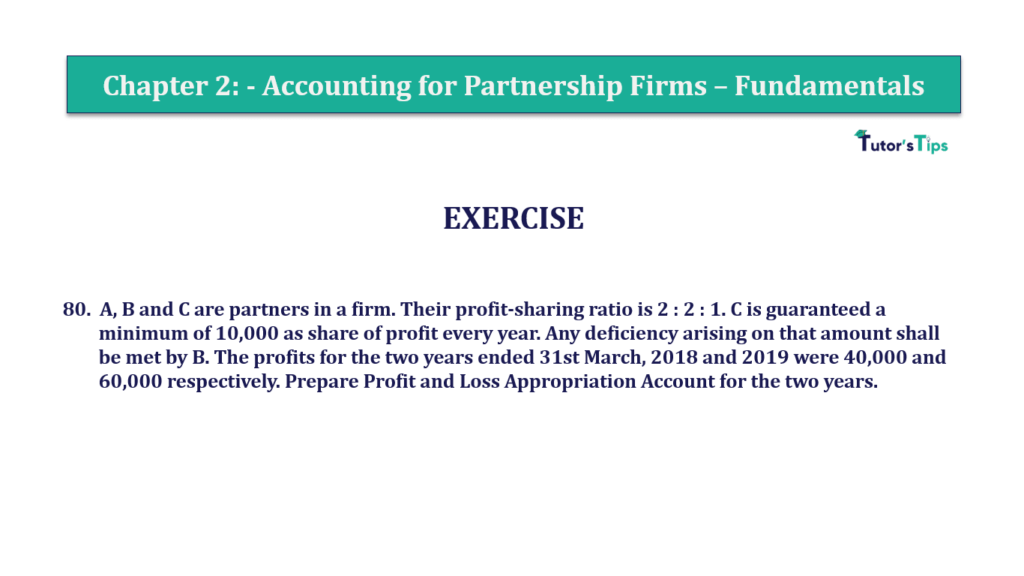# Question 80 Chapter 2 of +2-A – T.S. Grewal 12 Class Part – A Vol. 1Question 80 Chapter 2 of +2-A

80. A B and C are partners in a firm. Their profit-sharing ratio is 2: 2: 1. C is guaranteed a minimum of 10,000 as a share of profit every year. Any deficiency arising on that amount shall be met by B. The profits for the two years ended 31st March 2018 and 2019 were 40,000 and 60,000 respectively. Prepare Profit and Loss Appropriation Account for the two years.

The solution of Question 80 Chapter 2 of +2-A

:

 Balance Sheet (for the year ended 31st March 2019) Liabilities Amount Assets Amount By Profit and Loss A/c 40,000 To Profit Transferred to *2 A’s Capital A/c 16,000 B’s Capital A/c 14,000 C’s Capital A/c 10,000 40,000 40,000 40,000

 Balance Sheet (for the year ended 31st March 2019) Liabilities Amount Assets Amount By Profit and Loss A/c 60,000 To Profit Transferred to *2 A’s Capital A/c 24,000 B’s Capital A/c 24,000 C’s Capital A/c 12,000 60,000 60,000 60,000

Working Note: –

Calculation of Actual Amount of Interest on A’s, B’s, & C’s Capital
Interest on Capital= Opening Capital X Rate of Interest

 Interest on A’s Capital = 3,00,000 X 10 100

Interest on A’s Capital = 30,000/-

 Interest on B’s Capital = 2,00,000 X 10 100

Interest on B’s Capital = 20,000/-

 Interest on C’s Capital = 1,50,000 X 10 X 6 100 12

Since Z is admitted on 1st October 2018 and Profit is ascertained on March 31, 2019, therefore, interest on capital is calculated for 6 months

Interest on Z’s Capital = 7,500 /-

Calculation of distribution of Profits for the year 2017-18 the among the partners
Profit for 2018 = 40,000
Profit-sharing ratio = 2 : 2 : 1

C is given a guarantee of a minimum profit of Rs 10,000

 A’s Share of Profit = 40,000 X 2 5

A’s Share of Profit  = 16,000

 Y’s Share of Profit = 40,000 X 2 5

Y’s Share of Profit= 16,000

 C’s Share of Profit = 40,000 X 1 5

C’s Share of Profit= 8,000

C’s Actual Profit Share i. e. Rs 8,000 is less than his Minimum Guaranteed Profit i. e. Rs 10,000
Deficiency in C’s Profit Share= 10,000 − 8,000= Rs 2,000
This deficiency is to be borne by B because he personally guaranteed it.

Now, Final distributed among the partners

 A’s Share of Profit = 16,000 – 0 =16,000 B’s Share of Profit = 16,000 – 2,000 =14,000 C’s Share of Profit = 38,250 + 2,000 =10,000

Calculation of distribution of Profits for the year 2017-18 the among the partners
Profit for 2018 = 60,000
Profit-sharing ratio = 2 : 2 : 1

C is given a guarantee of a minimum profit of Rs 10,000

 A’s Share of Profit = 60,000 X 2 5

A’s Share of Profit  = 24,000

 B’s Share of Profit = 60,000 X 2 5

B’s Share of Profit = 24,000

 C’s Share of Profit = 60,000 X 2 5

C’s Share of Profit = 12,000

This deficiency(if any) is to be borne by B, but in the year 2017-18 there is no deficiency

Also, Check out the solved question of previous Chapters: –

### T.S. Grewal’s Double Entry Book Keeping +2 (Vol. I: Accounting for Not-for-Profit Organizations and Partnership Firms)

• Chapter No. 1 – Financial Statement of Not-For-Profit Organisations
• Chapter No. 2 – Accounting for Partnership Firms – Fundamentals
• Chapter No. 3 – Goodwill: Nature and Valuation
• Chapter No. 4 – Change in Profit-Sharing Ratio Among the Existing Partners
• Chapter No. 5 – Admission of a Partner
• Chapter No. 6 – Retirement/Death of a Partner
• Chapter No. 7 – Dissolution of a Partnership Firm

### T.S. Grewal’s Double Entry Book Keeping (Vol. II: Accounting for Companies)

• Chapter No. 1 – Financial Statements of a Company
• Chapter No. 2 – Financial Statement Analysis
• Chapter No. 3 – Tools of Financial Statement Analysis – Comparative Statements and Common- Size Statements
• Chapter No. 4 – Accounting Ratios
• Chapter No. 5 – Cash Flow Statement